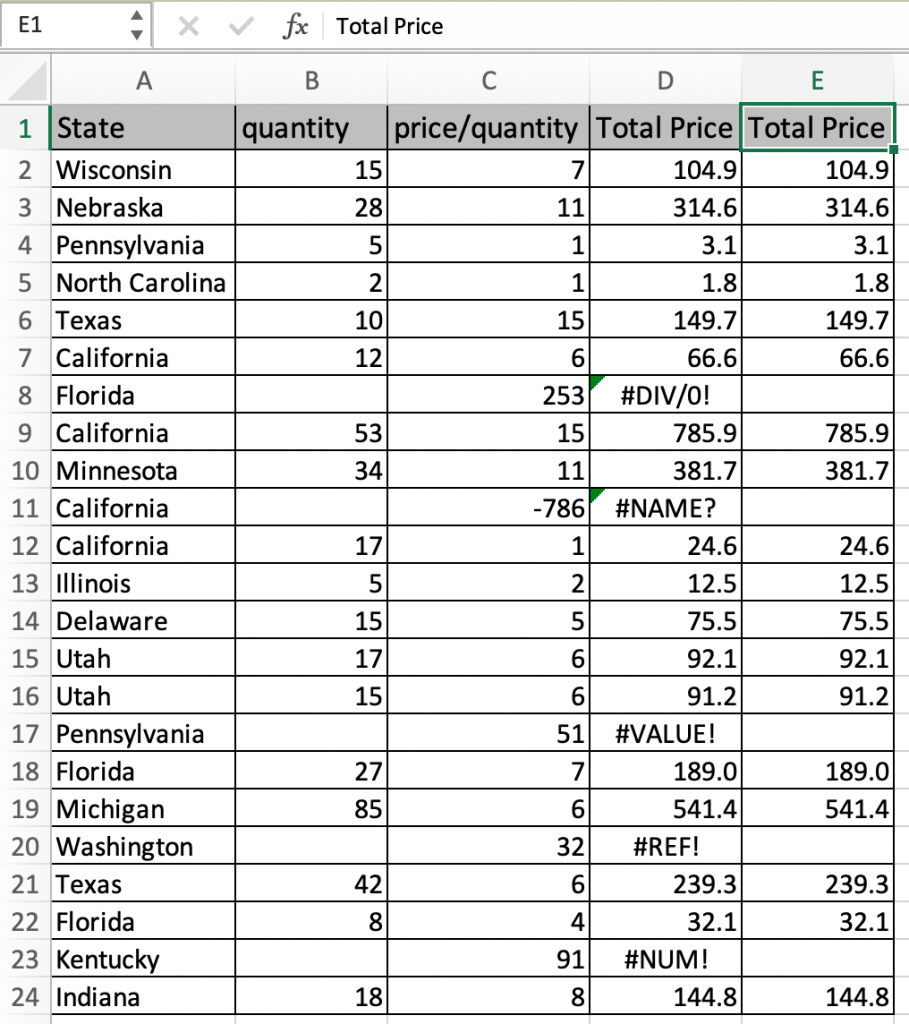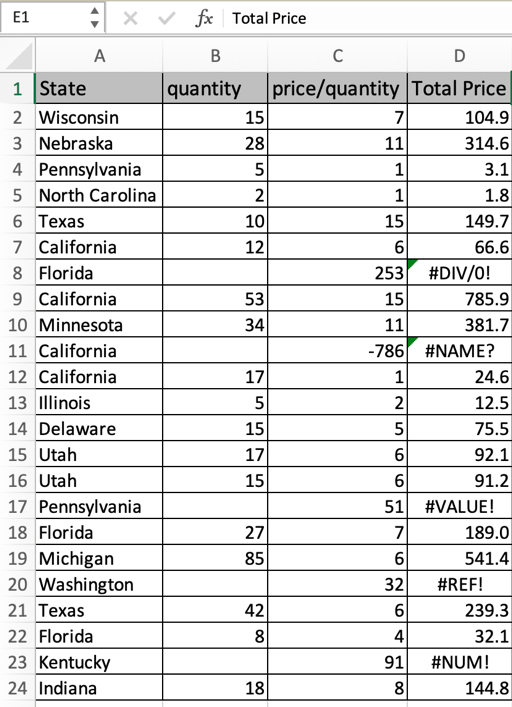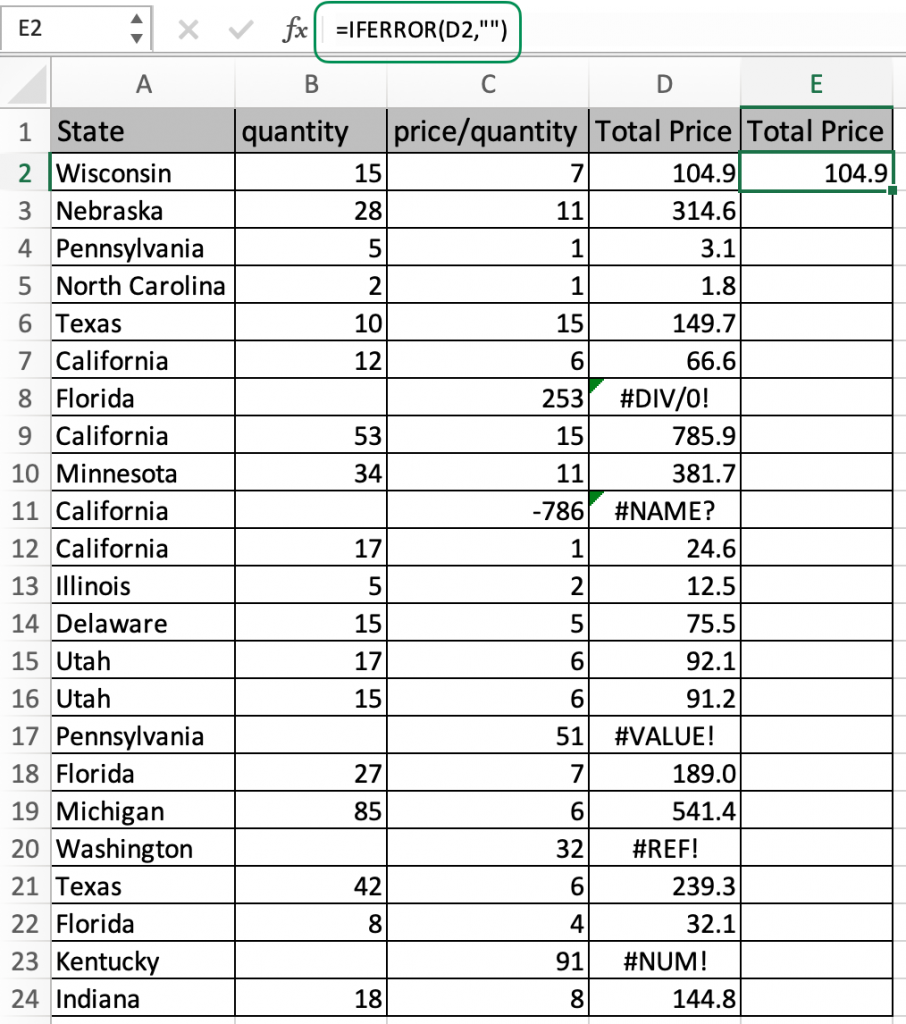# How to Hide errors before printing in ExcelIn this article, we will learn How to Hide errors before printing in Excel.

Scenario:

Errors in excel shows there is some problem in formula calculation, or misinterpretation of values. But sometimes you know errors are coming in data and doesn't need to print the data with error values. So for problems like these, there are many ways to handle it. But here we understand how we can do it easily using the IFERROR function. Let's learn about IFERROR function and then understand using an example.

IFERROR formula in Excel

IFERROR function takes the value and returns the value if the value is not error or if the value is error, then it returns the new value or blank, as it requires.

IFERROR formula syntax

 =IFERROR(cell_ref, value_if_error)

cell_ref : cell reference of the cell value to check

value_if_error : new value if cell contains error. Use "" if a blank cell is needed.

Alternate way

Use conditional formatting. Make a new rule with conditional formula =ISERROR(cell_ref) and select the font format as transparent or white. This will make the font disappear wherever the value is an error.

Example :

All of these might be confusing to understand. Let's understand how to use the function using an example. Here we have a table to print. The table is calculated using many formulas and has errors. But it doesn't look good with errors. We use blank cells.Make a new column Total price and use the formula in the E2 cell.

Use the formula:

 =IFERROR(D2,"")Copy and paste this formula to other cells using the Ctrl + D or dragging down from the tiny right bottom corner of the cell.As you can see all the errors are gone in the new column. Now hide the column with errors and print.

Alternate method

If there are few cells, then You can also just select the cell and switch the font color of cell value to transparent or white to make it invisible.

To make the above method interesting. We can use conditional formatting. Make a new rule with conditional formula =ISERROR(cell_ref) and now use the font format as transparent or white. Use this if you have a large amount of data with errors.

Here are all the observational notes using the formula in Excel
Notes :

1. The formula works with text and number both.
2. Use IFERROR while computing with other formulas. So the output of the formula is never an error.
3. There are 8 types of errors #DIV, #VALUE, #NUM, #NAME, #N/A, #REF, #null, #getting_data.

Hope this article about How to Hide errors before printing in Excel is explanatory. Find more articles on calculating formulas with error values and related Excel formulas here. If you liked our blogs, share it with your friends on Facebook. And also you can follow us on Twitter and Facebook. We would love to hear from you, do let us know how we can improve, complement or innovate our work and make it better for you. Write to us at info@exceltip.com.

Related Articles :

How to give the error messages in Data Validation : Restrict users to customize input information in the worksheet and guide the input information through error messages under data validation in Excel.

WHY #VALUE! Error occur And How to Fix It in Excel : Detect the unsupported variable in formula using the #VALUE! Error. Learn how to resolve different #VALUE! Error in Excel.

How to correct a #NUM! ErrorDetect the disallowed number with some functions like SQRT function using the #NUM! Error. Resolve this error using the ISERROR function to get more readable data in Excel.

Why #NAME? occur and How to fix #NAME? Error : Detect the unsupported or unrecognized keyword in formula using the #NAME? Error. Learn how to resolve different #NAME? Error in Excel.

Why #DIV/0! occur and How to fix #DIV/0! Error : Detect the mathematical errors like division by zero in formula using the #DIV/0! Error. Learn how to resolve different #DIV/0! Error in Excel.

Why #N/A occur and How to fix #N/A error : Detect the unavailability of value in dataset with formula using the #N/A Error. Resolve this error using the IFERROR or IFNA functions to get more readable data in Excel.

Why #NULL! occur and How to fix #NULL error : Detect the disallowed format in formula using the #NUM! Error. Learn the correct formula to resolve the #NULL Error in Excel.

Why #REF! occur and How to fix #REF! error : Detect the incorrect reference in formula using the #REF! Error. Learn the correct formula to resolve the #REF! Error in Excel.

Popular Articles :

How to use the IF Function in Excel : The IF statement in Excel checks the condition and returns a specific value if the condition is TRUE or returns another specific value if FALSE.

How to use the VLOOKUP Function in Excel : This is one of the most used and popular functions of excel that is used to lookup value from different ranges and sheets.

How to use the SUMIF Function in Excel : This is another dashboard essential function. This helps you sum up values on specific conditions.

How to use the COUNTIF Function in Excel : Count values with conditions using this amazing function. You don't need to filter your data to count specific values. Countif function is essential to prepare your dashboard.

Terms and Conditions of use

The applications/code on this site are distributed as is and without warranties or liability. In no event shall the owner of the copyrights, or the authors of the applications/code be liable for any loss of profit, any problems or any damage resulting from the use or evaluation of the applications/code.